Rounding Numbers

 Problem Set 1 Problem Set 2 Problem Set 3Select a problem set using the buttons above, then use your mouse or tab key to select a question. Fill in the blank with the correct answer for each problem. When you have answered all of the questions, ask Charlie how you did.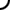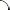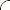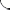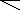Round each number as indicated. Use commas correctly.   Round 35 to the nearest ten. Round 243 to the nearest ten. Round 439 to the nearest hundred. Round 3,962 to the nearest hundred. Round 84,502 to the nearest thousand. Round 16,444 to the nearest ten thousand.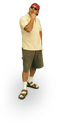Round each number as indicated. Use commas correctly.   Round 170 to the nearest ten. Round 5,497 to the nearest ten. Round 739 to the nearest hundred. Round 7,777 to the nearest hundred. Round 2,399 to the nearest thousand. Round 862,537 to the nearest ten thousand.Round each number as indicated. Use commas correctly.   Round 1,991 to the nearest ten. Round 4,327 to the nearest ten. Round 636 to the nearest hundred. Round 4,351 to the nearest hundred. Round 49,889 to the nearest thousand. Round 486,400 to the nearest ten thousand.©  2009  LarryPerezWeb Design:  Patrick Quigley